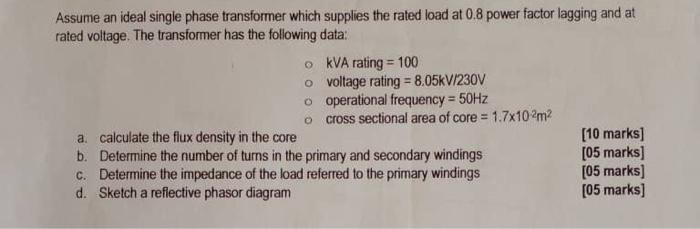# Question Assistance Assume an ideal single phase transformer which supplies the rated load at 0.8 power factor lagging and at rated voltage. The transformer has the following data: o kVA rating = 100 o voltage rating = 8.05kV/230V o operational frequency = 50Hz cross sectional area of core = 1.7x102m2 a calculate the flux density in the core [10 marks) b. Determine the number of turns in the primary and secondary windings [05 marks] c. Determine the impedance of the load referred to the primary windings [05 marks] d. Sketch a reflective phasor diagram [05 marks]PQJBSE The Asker · Electrical Engineering
AssistanceTranscribed Image Text: Assume an ideal single phase transformer which supplies the rated load at 0.8 power factor lagging and at rated voltage. The transformer has the following data: o kVA rating = 100 o voltage rating = 8.05kV/230V o operational frequency = 50Hz cross sectional area of core = 1.7x102m2 a calculate the flux density in the core [10 marks) b. Determine the number of turns in the primary and secondary windings [05 marks] c. Determine the impedance of the load referred to the primary windings [05 marks] d. Sketch a reflective phasor diagram [05 marks]
More
Transcribed Image Text: Assume an ideal single phase transformer which supplies the rated load at 0.8 power factor lagging and at rated voltage. The transformer has the following data: o kVA rating = 100 o voltage rating = 8.05kV/230V o operational frequency = 50Hz cross sectional area of core = 1.7x102m2 a calculate the flux density in the core [10 marks) b. Determine the number of turns in the primary and secondary windings [05 marks] c. Determine the impedance of the load referred to the primary windings [05 marks] d. Sketch a reflective phasor diagram [05 marks]